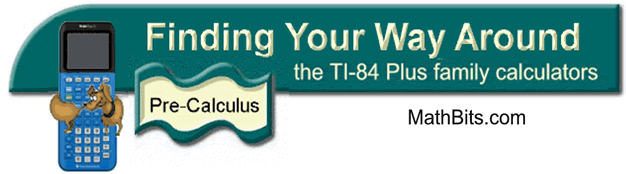Graphing Piecewise-Defined Functions

Piecewise-defined, or split definition, functions can be graphed on the graphing calculator.
You will need to utilize the relational operators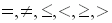found under the TEST menu (2nd MATH).

The format for entering these function can be either
(expression)/(condition)  or  (expression)*(condition)
where parentheses are essential in both situations.

Let's examine the differences between the two methods,
see how to enter compound inequalities (2 < x < 6),
and discover why these methods work.

 Method 1:  (expression)/(condition)

When using this method, enter each SECTION of the function into a separate Y= area.

Graph:Parentheses needed!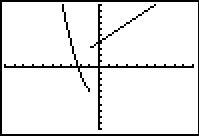If you use the TI-84+C, you can use the "fraction template" for the "pretty print" display.
Parentheses are not needed with the template. The fraction template is under ALPHA - F1.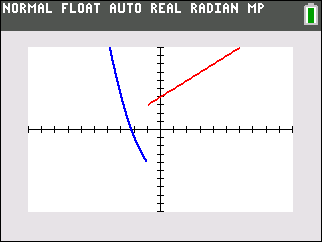NOTE:  Neither method will draw the "open" or "closed" circles at the endpoints of sections of the graph.  You will have to draw the appropriate circles when copying the graph. "open circle" for  < or > "closed circle" for < or >

 Advantages of this method: Disadvantages: 1.  This method of entering the function is helpful when trying to find any mistakes as each section can be altered independently. 1.  When using TRACE, you will need to use your up arrow to move between sections of the graph. 2.  The graph, while still in CONNECTED MODE, will not "connect" the separate sections together.  The graph will be a more realistic representation of the graph.

 Method 2:  (expression)*(condition)

When using this method, enter each SECTION of the function into a separate Y= area
OR enter the ENTIRE function as one statement using + sign to separate the sections.

 Graph: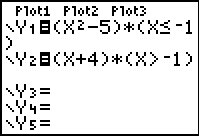Entered separately. OREntered as one statement
 The graphs from either of these entries will produce a connected graph. Unfortunately, DOT MODE is needed with this method to see the actual piecewise functional shape.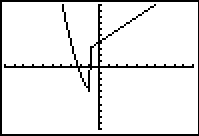Connected MODE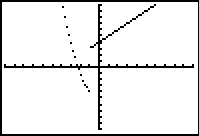DOT MODE
 Advantages of this method: Disadvantages: 1.  This method allows for the function to be entered as one statement.  When using TRACE, there will be no need to arrow up and down between sections of the graph. 1.  The graph, while still in CONNECTED MODE, will "connect" the separate sections together.  This can lead to confusion about the actual graph. 2.  This method requires a change to DOT MODE to get a realistic graph. 3.  Entering the function as one statement can be difficult to debug if an error occurs.

 Compound inequality:   -1 < x < 2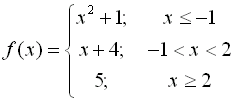Computers and calculators do not interpret expressions such as -1 < x < 2 as we do in mathematics.  Both computers and calculators see this expression as ONLY -1 < x (and they ignore the second part of the condition).  To force them to see the second condition, it it necessary to write the expression: (-1 < x < 2) as ((-1 < x) and (x < 2)) (Find and from the catalog or under TEST (2nd MATH) arrow right to LOGIC).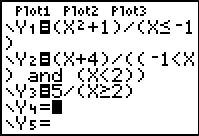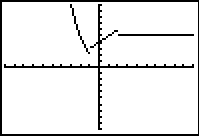In Connected MODEIf you use the TI-84+C, you can use the "fraction template" for the "pretty print" display.
Parentheses are not needed with the template. The fraction template is under ALPHA - F1.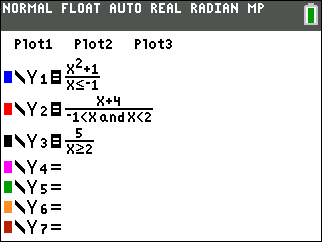So, what is really going on here???

How is this process working?

The calculator is doing a Boolean check on these conditions.  Remember, Boolean equates a true condition to be a 1 and a false condition to be a 0.

 Consider: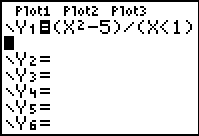When dealing with the division method, if a number is NOT in the interval, x < 1, the condition is assigned a value of 0, and the problem becomes division by zero.  Since this is not possible, an error is produced at those values and "nothing" is graphed in that area.  Since there is NO graph at these locations, there is also NO graphical connection being made, even though the calculator is in Connected MODE.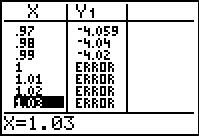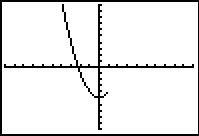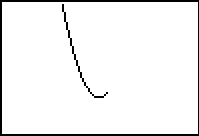Graph with axes turned off.

 When dealing with the multiplication method, if a number is NOT in the interval, x < 1, the condition is assigned a value of 0, and the problem becomes multiplication by zero.  Of course the "graphing" of this 0 value coincides with the x-axis and is not seen when the axes are visible.  Because this 0 value IS graphed, the Connected MODE kicks in, and the sections of the graph are connected together.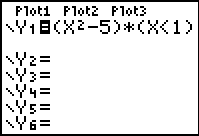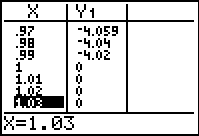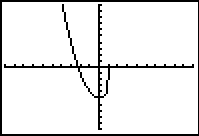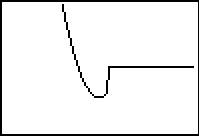Graph with axes turned off.